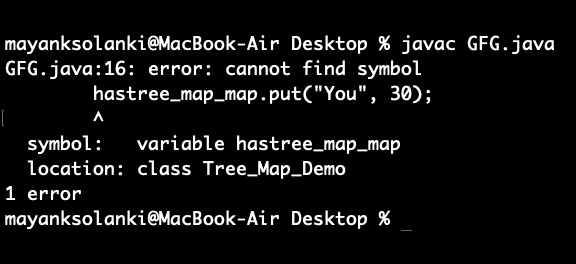GeeksforGeeks App
Open AppBrowser
Continue

# TreeMap containsValue() Method in Java With Examples

In Java, containsValue() method of the TreeMap class is used to check whether a particular value is being mapped by any key in the TreeMap. It takes the value as a parameter and returns True if that value is mapped by any of the keys in the map.

```--> java.util Package
--> TreeMap class
--> containsValue() Method  ```

Syntax:

`Tree_Map.containsValue(Object Value)`

Parameters: The value of Object type and refers to the value whose mapping is supposed to be checked by any key inside the map. ( Only one parameter)

Return Type: A boolean value, true if the mapping of the value is detected else false.

Note: In java, while returning boolean values are returned as true false only not as 0 and 1 as it is not allowed so do will throw error at compilation.

Example 1: Mapping String Values to Integer Keys.

## Java

 `// Java Program to Illustrate containsValue() Method``// of TreeMap class` `// Importing required classes``import` `java.util.*;` `// Main class``public` `class` `GFG {` `    ``// main driver method``    ``public` `static` `void` `main(String[] args)``    ``{``        ``// Creating an empty TreeMap by``        ``// declaring object of integer and string pairs``        ``TreeMap tree_map``            ``= ``new` `TreeMap();` `        ``// Mapping string values to int keys``        ``// using put() method``        ``tree_map.put(``10``, ``"Geeks"``);``        ``tree_map.put(``15``, ``"4"``);``        ``tree_map.put(``20``, ``"Geeks"``);``        ``tree_map.put(``25``, ``"Welcomes"``);``        ``tree_map.put(``30``, ``"You"``);` `        ``// Printing the elements of TreeMap``        ``System.out.println(``"Initial Mappings are: "``                           ``+ tree_map);` `        ``// Checking for custom value in map``        ``// using containsValue() method` `        ``// Say for the Value 'Geeks'``        ``System.out.println(``            ``"Is the value 'Geeks' present? "``            ``+ tree_map.containsValue(``"Geeks"``));` `        ``// Checking for the Value 'World'``        ``System.out.println(``            ``"Is the value 'World' present? "``            ``+ tree_map.containsValue(``"World"``));``    ``}``}`

Output

```Initial Mappings are: {10=Geeks, 15=4, 20=Geeks, 25=Welcomes, 30=You}
Is the value 'Geeks' present? true
Is the value 'World' present? false```

Example 2: Mapping Integer Values to String Keys.

## Java

 `// Java Program  to illustrate containsValue() Method``// of TreeMap class` `// Importing required classes``import` `java.util.*;` `// Main class``public` `class` `GFG {` `    ``// Main driver method``    ``public` `static` `void` `main(String[] args)``    ``{``        ``// Creating an empty TreeMap by``        ``// declaring object of string, integer pairs``        ``TreeMap tree_map``            ``= ``new` `TreeMap();` `        ``// Mapping int values to string keys``        ``// using put() method``        ``tree_map.put(``"Geeks"``, ``10``);``        ``tree_map.put(``"4"``, ``15``);``        ``tree_map.put(``"Geeks"``, ``20``);``        ``tree_map.put(``"Welcomes"``, ``25``);``        ``hastree_map_map.put(``"You"``, ``30``);` `        ``// Printing the elements of TreeMap``        ``System.out.println(``"Initial Mappings are: "``                           ``+ tree_map);` `        ``// Checking for the Value '10'``        ``// using containsValue() method``        ``System.out.println(``"Is the value '10' present? "``                           ``+ tree_map.containsValue(``10``));` `        ``// Checking for the Value '30'``        ``System.out.println(``"Is the value '30' present? "``                           ``+ tree_map.containsValue(``30``));` `        ``// Checking for the Value '40'``        ``System.out.println(``"Is the value '40' present? "``                           ``+ tree_map.containsValue(``40``));``    ``}``}`

Output:Note: Similarly the same operation can be performed with any type of Mappings with variation and combination of different data types.

My Personal Notes arrow_drop_up
Related Tutorials